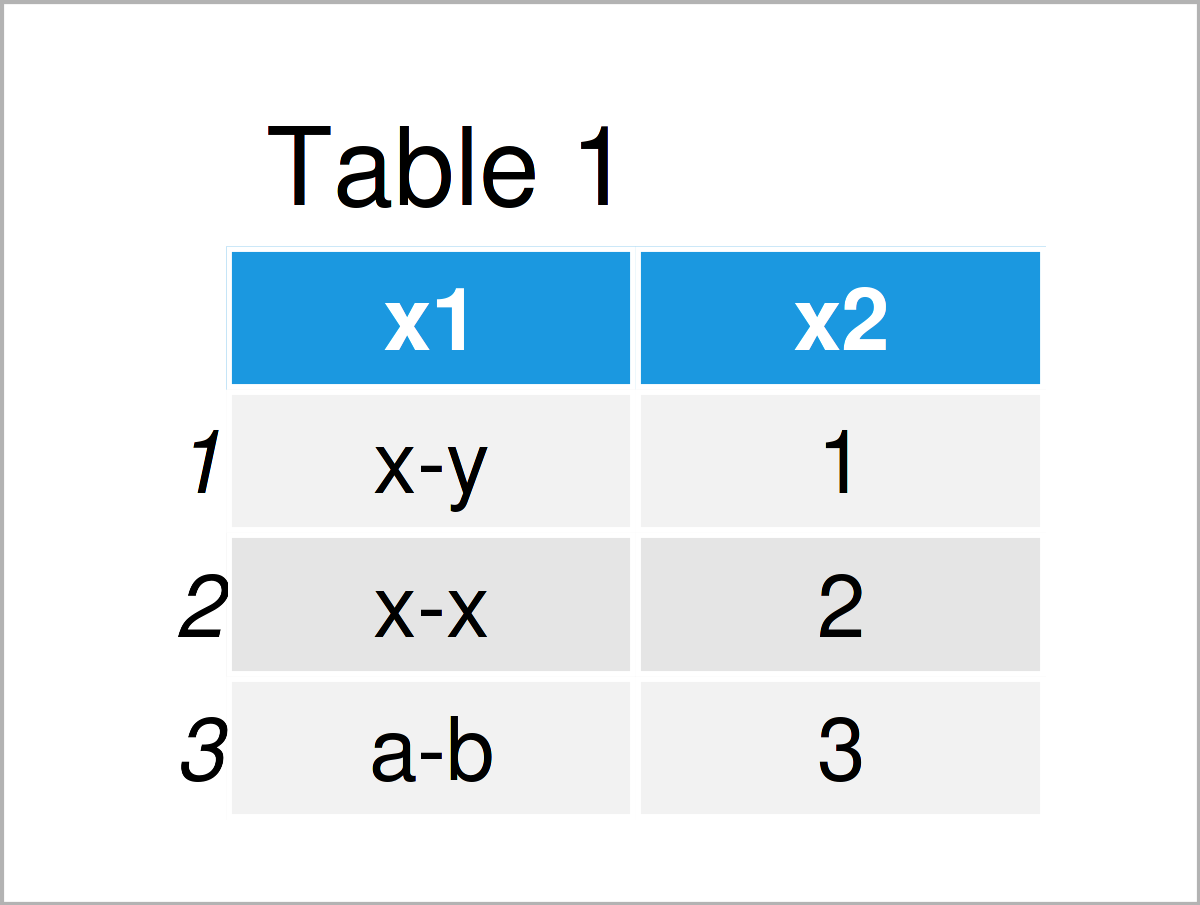# extract Function of tidyr Package in R (Example)

In this R tutorial you’ll learn how to split columns using the extract function of the tidyr package.

The article contains one example for the application of the extract function of the tidyr package. To be more specific, the article will contain this information:

Let’s start right away:

## Introducing Example Data

We’ll use the following data as basement for this R programming tutorial:

```data <- data.frame(x1 = c("x-y",             # Create example data
"x-x",
"a-b"),
x2 = 1:3)
data                                         # Print example data```Table 1 shows the structure of our example data – It consists of three rows and two variables. The variable x1 is a character and the variable x2 is an integer. Note that the variable x1 contains a – as separator between two letters.

## Example: Split Variable into Multiple Columns Using extract() Function of tidyr Package

In this section, I’ll explain how to use the extract function of the tidyr package to split data frame or tibble columns.

We first have to install and load the tidyr package, if we want to use the functions that are included in the package:

```install.packages("tidyr")                    # Install & load tidyr
library("tidyr")```

Next, we can apply the extract function to our example data frame as shown below:

```data_split <- tidyr::extract(data = data,    # Apply extract function
col = x1,
into = c("x1a", "x1b"),
regex = "([[:alnum:]]+)-([[:alnum:]]+)")
data_split                                   # Print new data```Table 2 illustrates our updated data set. As you can see, we have divided the variable x1 into two new variables based on the – sign as delimiter.

## Video & Further Resources

Do you need further info on the R programming syntax of this article? Then I recommend watching the following video on the Statistics Globe YouTube channel. I’m explaining the R programming code of this tutorial in the video:

Summary: In this R tutorial you have learned how to apply the extract function of the tidyr package. Let me know in the comments, if you have additional questions.

Subscribe to the Statistics Globe Newsletter